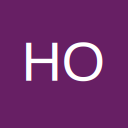Filter a Date column using VBA

• Hi,

I need to filter a Date column using VBA code and text box.

The user will type in the text box and the data will filter.

Below is the code I am using to filter columns that contain text data, and it works as intended:

Private Sub textbox_PanelDate_Change()
Dim S As String
S = textbox_PanelDate
If Len(S) > 0 Then
Range("B6").AutoFilter Field:=18, Criteria1:="*" & S & "*"
Else
Range("B6").AutoFilter Field:=18
End If
End Sub

What is the code to use to filter a column that contain date data?

Thank you.

• Hi,

I need to filter a Date column using VBA code and text box.

The user will type in the text box and the data will filter.

Below is the code I am using to filter columns that contain text data, and it works as intended:

Code
1. Private Sub textbox_PanelDate_Change() Dim S As String S = textbox_PanelDate If Len(S) > 0 Then Range("B6").AutoFilter Field:=18, Criteria1:="*" & S & "*" Else Range("B6").AutoFilter Field:=18 End IfEnd Sub

What is the code to use to filter a column that contain date data?

Thank you